Newton’s Law of Cooling

• Last Updated : 16 Jun, 2021

When hot water or milk is left on a table, it gradually cools. It eventually reaches the temperature of the surroundings. The hot water or milk can cool down by exchanging heat with the environment. Here, the cooling of hot water depends upon the difference between its temperature and the surroundings.

It is observed in the graph that the rate of cooling is faster at first and then slows as the body temperature drops. A hot body loses heat to its surroundings in the form of heat radiation. The rate of loss of heat depends on the difference in temperature between the body and its surroundings.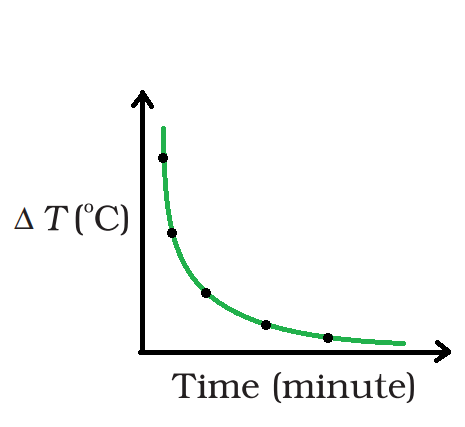A curve showing cooling of hot water with time

Newton’s Law of Cooling

Newton was the first to analyze the relationship between the heat lost by a body in a certain enclosure and its temperature systematically.

Newton’s law of cooling defines the rate at which an exposed body changes temperature by radiation, which is roughly equal to the difference in temperature between the item and its surroundings, provided the difference is small. However, take in mind that the difference over here is very small.

Newton’s law of cooling states that the rate of heat loss from a body is directly proportional to the difference in temperature between the body and its surroundings.

Using Newton’s rule of cooling, we can determine how quickly a substance at a given temperature will cool in any given environment. Furthermore, it explains how the rate of cooling of an object is affected not only by the temperature differential between the material and its surroundings but also by the cooling constant of the material.

Newton’s Law of Cooling Formula

Sir Isaac Newton, a renowned physicist, devised a formula for calculating the temperature of a material as it loses heat. Furthermore, the heat from the object is transferred to the surrounding environment. As previously stated, the rate of temperature change is related to the temperature differential between the item and its surroundings.

According to Newton’s law of cooling, the rate of loss of heat, that is – dQ/dt of the body is directly proportional to the difference of temperature is, ΔT = (T2 – T1) of the body and the surroundings.

The law is only valid for small temperature differences. Furthermore, the amount of heat lost through radiation is determined by the composition of the body’s surface and the extent of the exposed surface.

Therefore, the expression can be written as

– dQ/dt = k(T2 – T1)                                                                                                                                                                                            ……(1)

Here, k is a positive constant depending upon the area and nature of the surface of the body.

Derivation of Newton’s Law of Cooling

Let a body of mass m, with specific heat capacity s, is at temperature T2 and T1 is the temperature of the surroundings.

If the temperature falls by a small amount dT2 in time dt, then the amount of heat lost is,

dQ = ms dT2

The rate of loss of heat is given by,

dQ/dt = ms (dT2/dt)                                                                                                                                                                              ……..(2)

Compare the equations (1) and (2) as,

– ms (dT2/dt) = k (T2 – T1)

Rearrange the above equation as:

dT2/(T2–T1) = – (k / ms) dt

dT2 /(T2 – T1) = – Kdt

where K = k/m s

Integrating the above expression as,

loge (T2 – T1) = – K t + c

or

T2 = T1 + C’ e–Kt

where C’ = ec

The above expression is used to calculate the time of cooling of a body through a particular range of temperature.

The cooling curve is a graph that shows the relationship between body temperature and time. The rate of temperature fall is determined by the slope of the tangent to the curve at any point.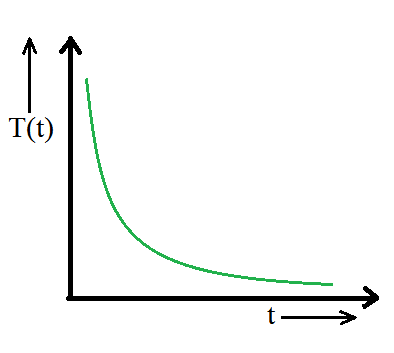The Cooling curve

In general, T(t) = TA+(TH-TA)e-kt

where T(t) is the Temperature at time t, TA is the Ambient temperature or temp of surroundings, TH is the temperature of the hot object, k is the positive constant and t is the time.

Methods to Apply Newton’s Law of Cooling

It is assumed that a constant rate of cooling, which is equal to the rate of cooling related to the average temperature of the body during the interval when rough values from Newton’s law are needed. i.e.

dθ\dt = k(<q> – q0)                                                                                                                                                                      ……..(3)

If qi is the initial temperature and qf is the final temperature of the body then,

<q> = (qi + qf)/2                                                                                                                                                                               ……….(4)

The above equation (4) is only an approximation or rough values and equation (3) is used for exact values of Newton’s Law of cooling.

The unknown parameter k may be computed using this type of cooling data, which may be monitored and visualized. In some cases, the parameter can be calculated numerically.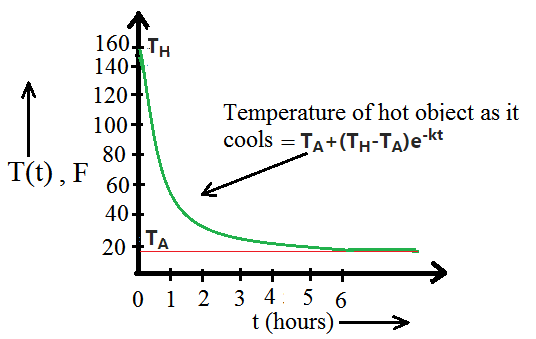An example showing temperature with time graph with data.

Verification of Newton’s Law of Cooling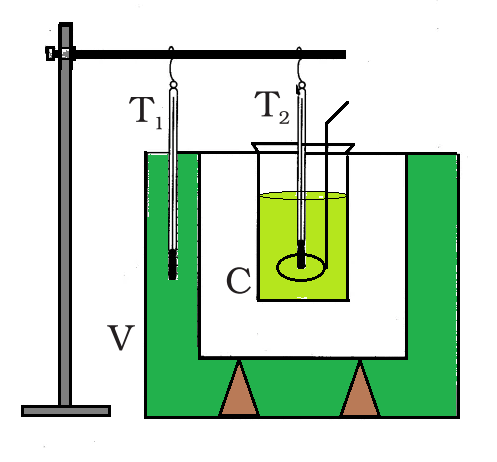Verification of Newton’s Law of cooling.

The experimental set-up presented in Figure can be used to verify Newton’s law of cooling. A double-walled vessel (V) with water in between the two walls makes up the setup. Inside the double-walled vessel is a copper calorimeter (C) containing hot water.

Two thermometers are used to note the temperatures T2 of the water in the calorimeter and T1 of the hot water between the double walls, respectively, through the corks. After equal intervals of time, the temperature of hot water in the calorimeter is recorded.

A graph between the loge (T2–T1) and time (t) appears as a straight line with a negative slope.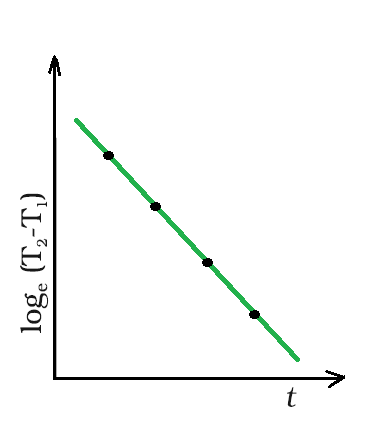The graph between the loge (T2–T1) and time (t)

Limitations of Newtons Law of cooling are:

• The temperature difference between the body and the environment must be small.
• Only radiation should be used as the loss of heat from the body.
• The temperature of the surroundings must remain constant during the cooling of the body, which is a key constraint of Newton’s law of cooling.

Applications of Newtons Law of cooling are:

• To estimate how long a warm object will take to cool down to a specific temperature.
• To determine the temperature of a drink in a refrigerator after a particular length of time has passed.
• It helps to indicate the time of death by looking at the possible body temperature at the time of death and the current body temperature.

Sample Problems

Problem 1: A pan filled with hot food cools from 94 °C to 86 °C in 2 minutes when the room temperature is at 20 °C. How long will it take to cool from 71 °C to 69 °C?

Solution:

The average temperature of 94 °C and 86 °C is 90 °C, which is 70 °C above the room temperature. Under these conditions the pan cools 8 °C in 2 minutes.

According to Newton’s law of cooling,

– dQ/dt = k(T2–T1

Substitute the value in the above expression,

8 °C /2 min = k(70 °C)                                                                                                                                                                    ………(1)

The average of 69 °C and 71 °C is 70 °C, which is 50 °C above room temperature. the value of K is the same.

Substitute the value in the above expression,

2 °C /dt = k(50°C)                                                                                                                                                                           ……(2)

Equate equation (1) and (2),

dt = 0.7 min

or time is equal to 42 s.

Problem 2: What is Newton’s Law of Cooling?

Solution:

Newton’s law of cooling states that the rate of heat loss from a body is directly proportional to the difference in temperature between the body and its surroundings.

According to Newton’s law of cooling, the rate of loss of heat, that is – dQ/dt of the body is directly proportional to the difference of temperature ΔT = (T2–T1) of the body and the surroundings. The law is only valid for small temperature differences. Furthermore, the amount of heat lost through radiation is determined by the composition of the body’s surface and the extent of the exposed surface.

Therefore, the expression can be written as

– dQ/dt = k(T2–T1

Problem 3: Why hot milk is easier to drink from a bowl than from a glass.

Solution:

Bowl has greater surface area than glass therefore the more heat  loses to its surroundings in the form of heat radiation through the bowl. The cooling of hot water depends upon the difference between its temperature and the surroundings. The rate of cooling is faster at first and then slows as the temperature drops.

Problem 4: Draw the graph that represents the transition of hot water to cool water.

Solution:

A curve showing cooling of hot water with time:A curve showing cooling of hot water with time

The cooling of hot water depends upon the difference between its temperature and the surroundings. It is observed in the graph that the rate of cooling is faster at first and then slows as the body temperature drops. A hot body loses heat to its surroundings in the form of heat radiation. The rate of loss of heat depends on the difference in temperature between the body and its surroundings.

Problem 5: A body at temperature 40ºC is kept in a surrounding of constant temperature 20ºC. It is observed that its temperature falls to 35ºC in 10 minutes. Find how much more time will it take for the body to attain a temperature of 30ºC.

Solution:

According to Newtons law of cooling

qf = qi e-kt

Now, for the interval in which temperature falls from 40 ºC to 35 ºC.

(35 – 20) = (40 – 20) e-(10k)

e-10k = 3/4

-10k = (ln 4/3)

k = 0.2876/10

k = 0.02876

Now, for the next interval;

(30 – 20) = (35 – 20)e-kt

10 = 15e-kt

e-kt = 2/3

-kt = ln(2/3)

t = 0.40546/k

Subtitte the value of k in the above equation,

t = 0.40546/0.02876

t = 14.098 min.

Problem 6: The oil is heated to 70 ºC. It cools to 50 ºC after 6 minutes. Calculate the time taken by the oil to cool from 50 ºC to 40 ºC given the surrounding temperature Ts = 25 ºC

Solution:

Given,

The temperature of oil after 6 min i.e. T(t) is equal to 50 ºC.

The ambient temperature Ts is   25 ºC.

The temperature of oil, To is 70 ºC.

The time to cool to 50ºC is 6 min.

According to Newton’s law of cooling,

T(t) = Ts + (T0 – Ts) e-kt

(T(t) – Ts)/(To – Ts) = e-kt

-kt = ln[(T(t) – Ts)/(To – Ts)]                                                                                                                                                        ………(1)

Substitute the above data in Newton’s law of cooling expression,

-kt = ln[(50 – 25)/(70 – 25)]

-k = (ln 0.55556)/6

k = 0.09796

The average temperature is equal to 45 ºC

Substitute the values in equation (1),

-(0.09796) t = ln[(45 – 25)/(70 – 25)]

-0.09796t = ln(0.44444)

0.09796t = 0.81093

t = 0.09796/0.58778 = 8.278 min.

Problem 7: Water is heated to 80 ºC for 10 min. How much would be the temperature in degree Celsius, if k = 0.056 per min and the surrounding temperature is 25 ºC?

Solution:

Given,

The ambient temperature Ts is 25 ºC,

The temperature of water T0 is 80 ºC.

The time that water is heated is t 10 min.

The value of constant k is 0.056.

According to Newton’s law of cooling,

T(t) = Ts + (T0 – Ts) e-kt

Substitute the above data in the above expression,

T(t)= 25 + (80 – 25)e-(0.056×10)

T(t) = 25+55 e-(0.056×10)

T(t) = 25+31.42

T(t) = 56.42

After 10 min the temperature cools down from 80 ºC to 56.42 ºC.

My Personal Notes arrow_drop_up